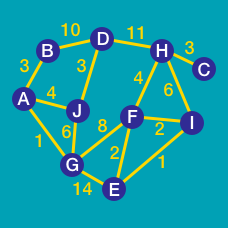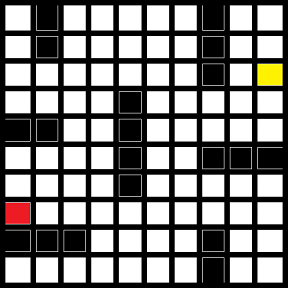Computer Science

# A* search

Consider the given maze below. You want to get from the red dot to the yellow dot. What is the length of the path found by an A* search that uses a Euclidean distance heuristic?The 8-puzzle is a sliding puzzle that consists of a frame of numbered square tiles in random order with one tile missing. The goal is to slide the tiles until the whole puzzle is in order.It turns out that A* can be used to solve this puzzle. If we represent a certain configuration of the board as a node, we can say its neighboring nodes are the 2-4 possible states achievable by moving the empty space. Thus the problem is reduced to a path finding problem. Our goal state is of-course the solved 8-puzzle.(The puzzle on the right in the image above)

Suppose we use the following heuristic for finding $h$. The heuristic is a simple function $h = \sum d(x_i)$ where $d(x)$ is the Manhattan distance of each square $x_i$ from its goal state.

For example for the start state in the image above , $h = 1 + 1 + 1 + 3 + 1 + 3 + 3 + 2 = 15$

Suppose we solve the same start state using this heuristic coupled with A* search. How many moves would it take to solve the puzzle?

Consider the given maze below. You want to get from the red dot to the yellow dot. What is the length of the path found by an A* search that uses a Manhattan distance heuristic?Details and assumptions

• The length is simply the number of cells that constitute the path. This includes the start and ending cells.
×